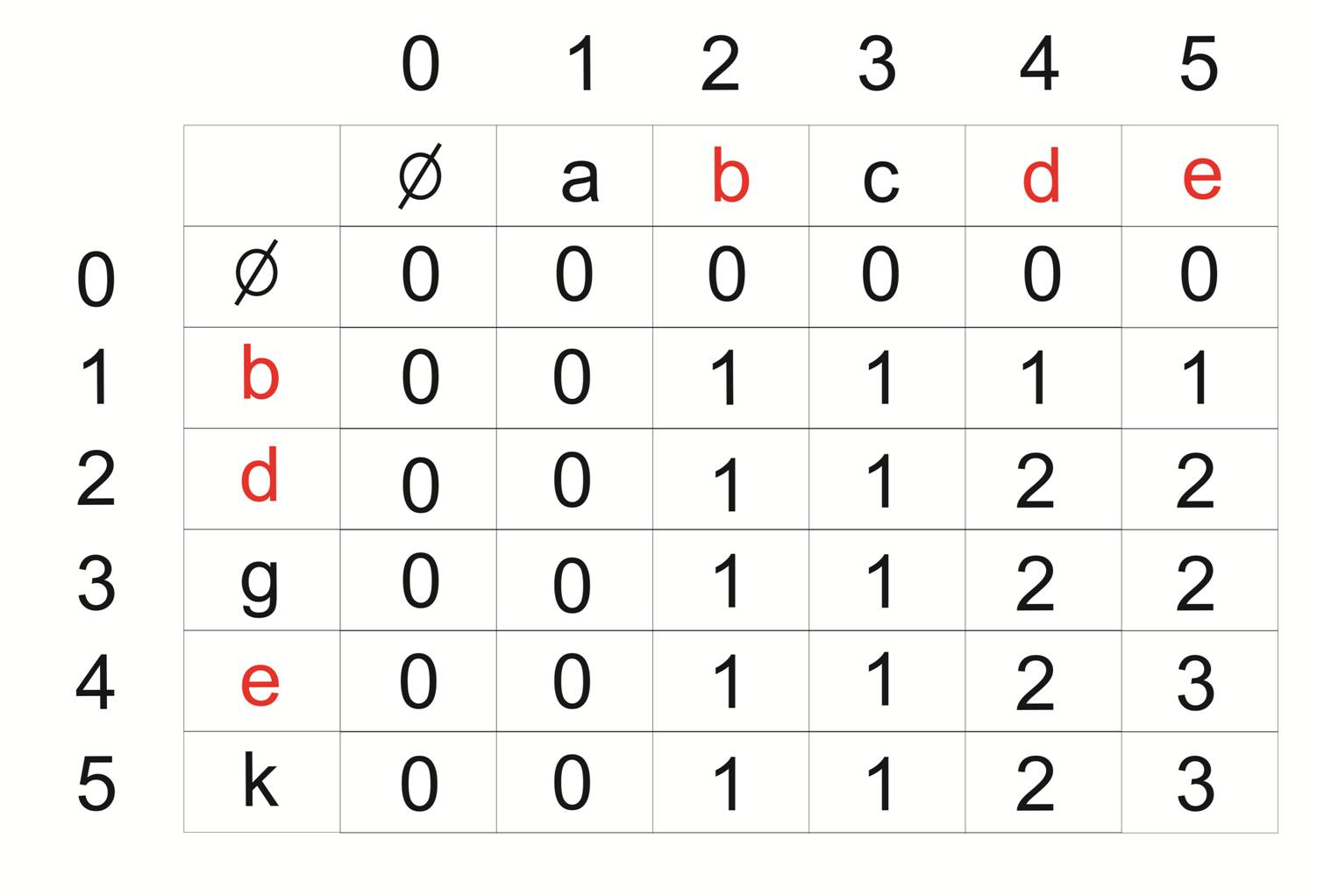# Print Longest common subsequence

INPUT
s1 = “abcde”
s2 = “bdgek”

OUTPUT
LCS is “bde”

## Algorithm

1. Build L[m+1][n+1], where L[i][j] contains length of LCS of s1[0…i-1] and s2[0…j-1]

a. Simply run two loops, outer loop variable i, inner loop variable j
b. For each character in s1(outer loop) select all the characters in s2(inner loop)
c. If  i == 0 and j == 0, then make L[i][j] =0 //Base case
d. If s1[i-1] == s2[j-1], then make L[i][j] = L[i-1][j-1] + 1
e. else, L[i][j] = max(L[i-1][j], L[i][j-1])

L[][] for above example.2. As we can see L[m][n] contains the length of the longest common subsequence. create a character array LCS[] to print the longest common subsequence

3. Traverse the array L[m][n]

a. if s1[i-1] == s2[j-1], then include this character in LCS[]
b. else, compare values of L[i-1][j] and L[i][j-1] and go in direction of greater value.

## C++ Program

```#include <bits/stdc++.h>
using namespace std;

//Prints the Longest common subsequence
void printLCS( char *s1, char *s2, int m, int n )
{
int L[m+1][n+1];

//Building L[m][n] as in algorithm
for (int i=0; i<=m; i++)
{
for (int j=0; j<=n; j++)
{
if (i == 0 || j == 0)
L[i][j] = 0;
else if (s1[i-1] == s2[j-1])
L[i][j] = L[i-1][j-1] + 1;
else
L[i][j] = max(L[i-1][j], L[i][j-1]);
}
}

//To print LCS
int index = L[m][n];
//charcater array to store LCS
char LCS[index+1];
LCS[index] = '\0'; // Set the terminating character

//Stroing characters in LCS
//Start from the right bottom corner character
int i = m, j = n;
while (i > 0 && j > 0)
{
//if current character in s1 and s2 are same, then include this character in LCS[]
if (s1[i-1] == s2[j-1])
{
LCS[index-1] = s1[i-1]; // Put current character in result
i--; j--; index--;     // reduce values of i, j and index
}
// compare values of L[i-1][j] and L[i][j-1] and go in direction of greater value.
else if (L[i-1][j] > L[i][j-1])
i--;
else
j--;
}

// Print the LCS
cout << "LCS of " << s1 << " and " << s2 << " is "<<endl << LCS<<endl;
}

/* Driver program to test above function */
int main()
{
char s1[] = "abcde";
char s2[] = "bdgek";
printLCS(s1, s2, strlen(s1), strlen(s2));
return 0;
}```

Translate »